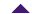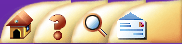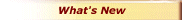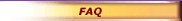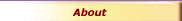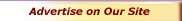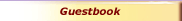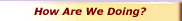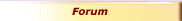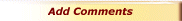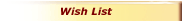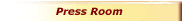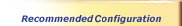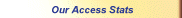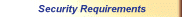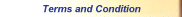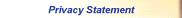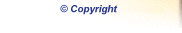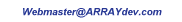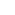Q. What exactly does DeLiberation™ Financial Calculator calculate?
 A. The DeLiberation™ Financial Calculator has two principal functions. It can calculate the growth of savings and investments with time. As well, it can calculate payments for interest loans.Q. Who could use this Calculator?
 A. DeLiberation™ Financial Calculator could be used by clients of financial institutions that offer lending and investment services. It could also be used by business and accounting experts, consumers of merchandise offered under leasing or financing arrangements, and real estate buyers (e.g., to compare mortgage and rent payments).Q. How does DeLiberation™ Financial Calculator differ from other Internet calculators?
 A. DeLiberation™ Financial Calculator has been designed to offer maximum flexibility in interest calculations. Interest can be calculated on a regular or compound basis, and compounded annually, quarterly, monthly, weekly, bi-weekly, daily, hourly or even by the minute. Moreover, our Calculator allows the interest rate to fluctuate throughout the capitalization period. Unlike most calculators on the Internet, DeLiberation™ Financial Calculator is not a client-executed application. Since the program does not have to be downloaded into the user's computer, there is a faster loadtime and less danger of security breaches. Moreover, its use is not limited by the user's hardware, operating system or browser.Q. What is the difference between simple and compound interest?
 A. Simple interest is calculated just on the initial amount. Compound interest is calculated on the initial amount plus the interest earned at a given interval.Q. What is the difference between fixed and floating interest rates?
 A. Fixed interest remains constant throughout the investment or borrowing period. Floating interest occurs when the rate fluctuates.Q. What is the difference between nominal and effective rates?
 A. A nominal rate is the "raw" value that is used to compute interest. An effective rate represents the "true" amount that the principal grows by. For example, if a nominal rate were defined as 10% compounded annually, but there were monthly contributions ... then the calculator would actually compound at 0.10/12 or 0.833% monthly, to account for the contributions. Due to the nature of compounding, it actually amounts to a growth of 10.47% annually. If the same scenario were considered with a 10% effective annual rate, then the monthly compounding rate would only be 0.797%. It would result in the principal growing by exactly 10% annually.Q. Do periodic contributions include an initial deposit of the desired amount?
 A. No initial deposit is being considered for periodic contributions. The initial amount is the value entered in the Principal window. All contributions are assumed to be made at the end of each contribution period.Q. How do I suggest further improvements to the DeLiberation™ Financial Calculator?
 A. Please do provide your suggestions to our developers by filling out a Questionnaire or by leaving a message in our Guestbook.Q. Can your calculator be integrated into our Web site or is it designed as a "link-to" solution?
 A. Our calendar is designed as a customized module to be brandlined and integrated into your Web site. Of course we can arrange its hosting on another reliable Web hosting service, if that's what you would prefer.Q. What is the license cost?